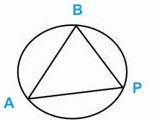Question: What is Subtend an Angle ? Answer: To subtend an angle is to form an angle at a point. When you look at an object such as a tree, the angle subtended at your eye is that formed by imagining two lines drawn from the top and bottom of the object to your eye.When two lines AP and BP are drawn joining the three points A, B and P on a circle, then the angle APB is said to be subtended by the chord AB or by the arc AB. All the angles formed on one side of a chord of a circle in this way are equal to each other. The angles subtended by a diameter at its circumference are all 90o.